Next: About this document ... Up: lab_template Previous: lab_template

Subsections

# More on Differentiation

## Background

Maple knows how to take many derivatives. Its main commands for doing this are D and diff. D is designed to differentiate functions, whereas diff is for differentiating expressions. However, if proper notation is used, diff can also be used with functions. To review the difference between a function and an expression, check the two examples below. The f statement defines a function, the g statement defines an expression.
> f:=x->x^2+7*x+5;
> g:=x^3-5*x+8;

Here are some examples that show how D and diff work. Check the difference between these two commands.
> D(f)(x);
> diff(g,x);
> diff(f(x),x);

See what happens when the function or expression notations are used incorrectly.
> diff(g(x),x);
> diff(f,x);

After the last four examples, you should be convinced that proper notation is very important in doing derivatives in Maple. Maple can also do higher derivatives. Check these commands. Again, pay attention to the difference between expressions and functions.
> diff(g,x,x);
> diff(g,x,x,x);
> (D@@2)(f)(x);
> (D@@3)(f)(x);

If you want to evaluate the higher derivative at a specific value of x, you can use the following
> (D@@2)(f)(2);
> subs(x=3,diff(g,x));

Suppose you wanted to find the equation of the tangent line to the graph of f(x) at the point x = 5. This can be done in Maple using the point-slope form of a line as shown below. It is not necessary to label the command tanline; but giving the line a name makes it can easy to call it up if it is needed later.
> tanline := D(f)(5)*(x-5)+f(5);

More information on D and diff can be obtained through Maple help screens.

## Implicit Differentiaition

The implicitdiff command can be used to find derivatives of implicitly defined functions. The syntax is as follows
> f:=x^2*y^2+y^3=0;
> implicitdiff(f,y,x);

where f is an equation, y is the dependent variable and x is the independent variable. Thus the command as just stated would compute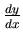. If f is given as an expression Maple will assume the implicit equation is f = 0. Check the results of the following commands.
> g:=x^2+y^3=1;
> implicitdiff(g,y,x);

Second derivatives can also be taken with implicitdiff. The following command computes.
> implicitdiff(g,y,x,x);

Maple also has a command for plotting implicitly defined functions. It is in the package plots which must be called before using the command.
> with(plots):
> implicitplot(x^2-y^2=1,x=-3..3,y=-3..3);

Suppose you want to find the equation of the line tangent to the graph of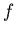defined implicitly. For instance, find the equation of the line tangent to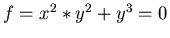at the point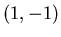. You can use the point-slope form of a line in implicit form to get the equation of the tangent line. The Maple commands below show how this can be done.
> f:=x^2*y^2+y^3=0;
> m:=subs({x=1,y=-1},implicitdiff(f,y,x));
> tanline := y-(-1)=m*(x-1);
> implicitplot({f,tanline},x=0..2,y=-3..0);


Sometimes you want the value of a derivative, but first have to find the coordinates of the point. More than likely, you will have to use the solve or fsolve command for this. However, to get the fsolve command to give you the solution you want, you often have to specify a range for the variable. Plotting the graph of a relation can be a big help in this task. For instance, if you wanted the slope ofas in the previous example atand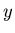is negative, but thevalue is not given, then you would first need to solve forby substituting the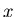value intoand then solve for. See how this is done below.

> f:=x^2*y^2+y^3=0;
> solve(subs(x=1,f),y);


## Exercises

1. Given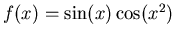, evaluate the third derivative atusing function notation and
1. the D command.
2. the diff command.

2. Find the equation of the line tangent to the graph ofat the point where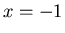andis positive. Include a plot of the relation and the tangent line on the same graph over the interval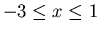and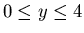.

3. Consider the graph defined implicitly by the equation.
1. Enter the equation, calling it h.
2. Use the implicitplot command to verify visually that the graph is an ellipse.
3. Find the slopes to this curve at the two points where it intersects the x-axis labeling them m1 and m2. (Hint: You will first need to use the solve command to find the y-values) What can you tell about the tangent lines at these points given your answers for the slopes?
4. Find the equations of the tangent lines to this curve at the two points where it intersects the x-axis labeling them t1 and t2. Remenber to enter the equations implicitly.
5. Graph the ellipse and the two tangent lines on one graph.Next: About this document ... Up: lab_template Previous: lab_template
Dina Solitro
2005-11-16